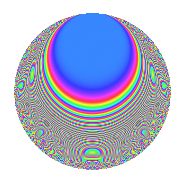# Properties

 Label 109.2.hLevel 109 Weight 2 Character orbit h Rep. character $$\chi_{109}(4,\cdot)$$ Character field $$\Q(\zeta_{18})$$ Dimension 48 Newforms 1 Sturm bound 18 Trace bound 0

# Related objects

## Defining parameters

 Level: $$N$$ = $$109$$ Weight: $$k$$ = $$2$$ Character orbit: $$[\chi]$$ = 109.h (of order $$18$$ and degree $$6$$) Character conductor: $$\operatorname{cond}(\chi)$$ = $$109$$ Character field: $$\Q(\zeta_{18})$$ Newforms: $$1$$ Sturm bound: $$18$$ Trace bound: $$0$$

## Dimensions

The following table gives the dimensions of various subspaces of $$M_{2}(109, [\chi])$$.

Total New Old
Modular forms 60 60 0
Cusp forms 48 48 0
Eisenstein series 12 12 0

## Trace form

 $$48q$$ $$\mathstrut -\mathstrut 9q^{2}$$ $$\mathstrut -\mathstrut 6q^{3}$$ $$\mathstrut +\mathstrut 15q^{4}$$ $$\mathstrut -\mathstrut 6q^{5}$$ $$\mathstrut +\mathstrut 3q^{7}$$ $$\mathstrut +\mathstrut 12q^{9}$$ $$\mathstrut +\mathstrut O(q^{10})$$ $$48q$$ $$\mathstrut -\mathstrut 9q^{2}$$ $$\mathstrut -\mathstrut 6q^{3}$$ $$\mathstrut +\mathstrut 15q^{4}$$ $$\mathstrut -\mathstrut 6q^{5}$$ $$\mathstrut +\mathstrut 3q^{7}$$ $$\mathstrut +\mathstrut 12q^{9}$$ $$\mathstrut -\mathstrut 9q^{10}$$ $$\mathstrut +\mathstrut 9q^{11}$$ $$\mathstrut -\mathstrut 3q^{12}$$ $$\mathstrut -\mathstrut 15q^{14}$$ $$\mathstrut -\mathstrut 12q^{15}$$ $$\mathstrut -\mathstrut 3q^{16}$$ $$\mathstrut -\mathstrut 9q^{17}$$ $$\mathstrut -\mathstrut 69q^{18}$$ $$\mathstrut -\mathstrut 9q^{19}$$ $$\mathstrut -\mathstrut 42q^{20}$$ $$\mathstrut +\mathstrut 15q^{21}$$ $$\mathstrut +\mathstrut 12q^{22}$$ $$\mathstrut -\mathstrut 18q^{23}$$ $$\mathstrut +\mathstrut 60q^{24}$$ $$\mathstrut -\mathstrut 18q^{25}$$ $$\mathstrut -\mathstrut 12q^{26}$$ $$\mathstrut -\mathstrut 45q^{27}$$ $$\mathstrut -\mathstrut 54q^{28}$$ $$\mathstrut +\mathstrut 33q^{29}$$ $$\mathstrut -\mathstrut 33q^{30}$$ $$\mathstrut -\mathstrut 18q^{31}$$ $$\mathstrut +\mathstrut 9q^{32}$$ $$\mathstrut +\mathstrut 3q^{34}$$ $$\mathstrut +\mathstrut 18q^{35}$$ $$\mathstrut +\mathstrut 105q^{36}$$ $$\mathstrut +\mathstrut 15q^{37}$$ $$\mathstrut -\mathstrut 33q^{38}$$ $$\mathstrut -\mathstrut 6q^{39}$$ $$\mathstrut -\mathstrut 12q^{40}$$ $$\mathstrut -\mathstrut 36q^{42}$$ $$\mathstrut +\mathstrut 9q^{43}$$ $$\mathstrut +\mathstrut 66q^{44}$$ $$\mathstrut -\mathstrut 6q^{45}$$ $$\mathstrut -\mathstrut 60q^{46}$$ $$\mathstrut -\mathstrut 6q^{47}$$ $$\mathstrut +\mathstrut 57q^{48}$$ $$\mathstrut +\mathstrut 39q^{49}$$ $$\mathstrut +\mathstrut 87q^{50}$$ $$\mathstrut -\mathstrut 48q^{51}$$ $$\mathstrut -\mathstrut 36q^{52}$$ $$\mathstrut +\mathstrut 15q^{53}$$ $$\mathstrut +\mathstrut 90q^{54}$$ $$\mathstrut -\mathstrut 9q^{55}$$ $$\mathstrut +\mathstrut 3q^{56}$$ $$\mathstrut +\mathstrut 15q^{57}$$ $$\mathstrut -\mathstrut 27q^{58}$$ $$\mathstrut -\mathstrut 6q^{59}$$ $$\mathstrut -\mathstrut 33q^{60}$$ $$\mathstrut +\mathstrut 78q^{61}$$ $$\mathstrut +\mathstrut 36q^{62}$$ $$\mathstrut +\mathstrut 72q^{63}$$ $$\mathstrut +\mathstrut 126q^{64}$$ $$\mathstrut -\mathstrut 54q^{65}$$ $$\mathstrut +\mathstrut 9q^{66}$$ $$\mathstrut +\mathstrut 54q^{67}$$ $$\mathstrut -\mathstrut 3q^{69}$$ $$\mathstrut +\mathstrut 39q^{70}$$ $$\mathstrut +\mathstrut 15q^{71}$$ $$\mathstrut -\mathstrut 99q^{72}$$ $$\mathstrut -\mathstrut 21q^{73}$$ $$\mathstrut +\mathstrut 24q^{74}$$ $$\mathstrut -\mathstrut 30q^{75}$$ $$\mathstrut +\mathstrut 81q^{77}$$ $$\mathstrut +\mathstrut 27q^{78}$$ $$\mathstrut -\mathstrut 126q^{79}$$ $$\mathstrut +\mathstrut 45q^{80}$$ $$\mathstrut -\mathstrut 75q^{81}$$ $$\mathstrut -\mathstrut 3q^{82}$$ $$\mathstrut -\mathstrut 21q^{83}$$ $$\mathstrut -\mathstrut 63q^{84}$$ $$\mathstrut -\mathstrut 6q^{85}$$ $$\mathstrut -\mathstrut 189q^{86}$$ $$\mathstrut +\mathstrut 114q^{87}$$ $$\mathstrut +\mathstrut 12q^{88}$$ $$\mathstrut +\mathstrut 3q^{89}$$ $$\mathstrut +\mathstrut 225q^{90}$$ $$\mathstrut -\mathstrut 72q^{91}$$ $$\mathstrut -\mathstrut 27q^{92}$$ $$\mathstrut +\mathstrut 33q^{93}$$ $$\mathstrut -\mathstrut 42q^{94}$$ $$\mathstrut -\mathstrut 39q^{95}$$ $$\mathstrut -\mathstrut 99q^{96}$$ $$\mathstrut +\mathstrut 51q^{97}$$ $$\mathstrut -\mathstrut 150q^{98}$$ $$\mathstrut -\mathstrut 93q^{99}$$ $$\mathstrut +\mathstrut O(q^{100})$$

## Decomposition of $$S_{2}^{\mathrm{new}}(109, [\chi])$$ into irreducible Hecke orbits

Label Dim. $$A$$ Field CM Traces $q$-expansion
$$a_2$$ $$a_3$$ $$a_5$$ $$a_7$$
109.2.h.a $$48$$ $$0.870$$ None $$-9$$ $$-6$$ $$-6$$ $$3$$